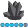## How can I add my own labels to a pie chart?

I want to replace the labels created by the slice= & percent= options of proc gchart.  I tried to annotate, but I am struggling.  Can anyone provide some examples please.   The chart is pretty simple and basic.

proc gchart data =chart1;

pie product_type / sumvar=trend_units type=sum nolegend slice=arrow percent=arrow value=none other=2 otherlabel="Other" coutline=black othercolor=red

annotate=anno

midpoints="Notebook" "Tablet" "Desktop" "AIO" "Monitor" "Dock" "Accessory" "Networking";

run;

quit;AncaTilea
Pyrite | Level 9

## Re: How can I add my own labels to a pie chart?

Hi,

What would you replace the labels with?

And what is your annotate code?

Here is an example of how to annotate stuff:

proc freq data = sashelp.class;

tables sex/out = freq;

run;

data anno;

length text \$14.;

set freq;/*(rename=(parameter=midpoint));*/;

retain xsys ysys "2" hsys "3" when "a" size;

function = "label"; style = '"Times New Roman/bold"' ;

position = "b"; size = 3.2 ;

if sex = "F" then do;

text = compress(put(percent, 4.2)||"%"); y = 60;x = 70;color = "green";

end;

if sex = "M" then do;

text = compress(put(percent, 4.2)||"%"); y = 40;x = 40;color = "red";

end;

output;

run;

proc gchart data = sashelp.class;

pie sex / anno = anno sumvar=weight ;

run;

quit;

Also,

here is a link to examples of pie charts:

Support.sas.com

Added some code and a link Message was edited by: Anca tilea

Discussion stats